# Basics of Time Intelligence in DAX for Power BI; Year to Date, Quarter to Date, Month to Date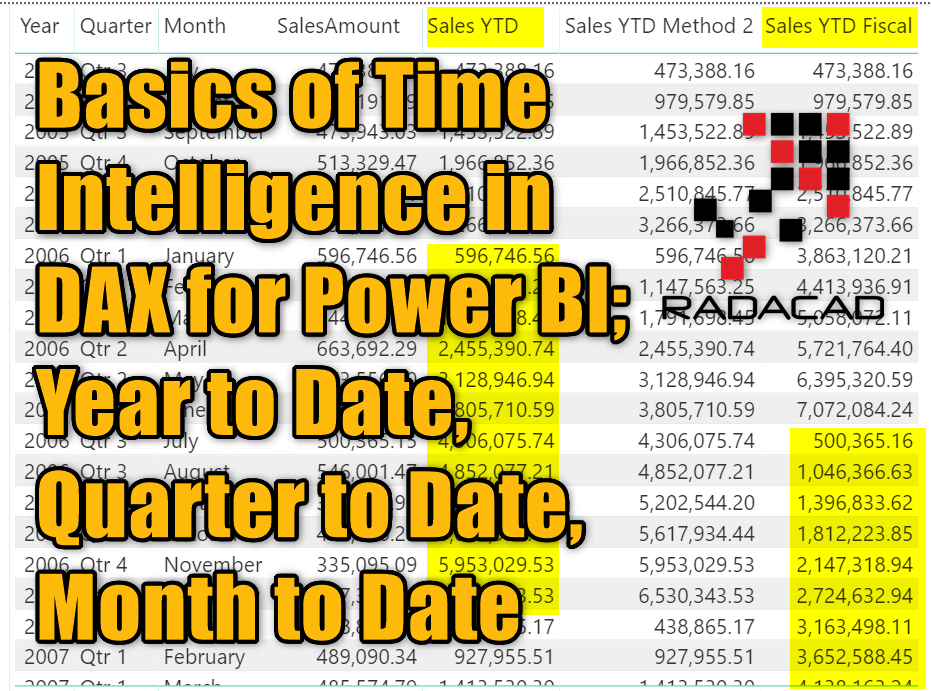#### Prerequisite

To run examples of this article, you would need to have AdventureWorksDW dataset. and the table we are using is only one table: FactInternetSales to be loaded into Power BI.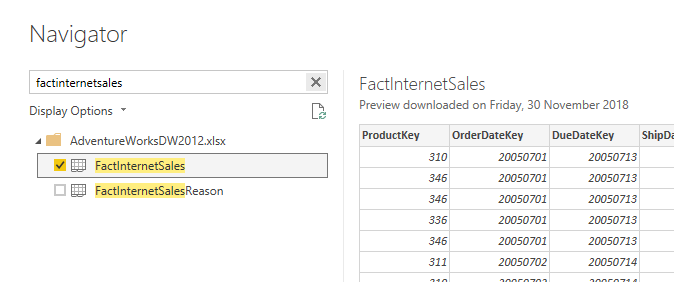## What is Time Intelligence?

Time Intelligence functions in DAX are a set of functions that give you insight from the date and time dimensions. Most of the analysis by date and time are in that category, as an example; year to date, quarter to date, month to date, same period last year calculations and etc. These calculations they all have one dimension in common; date/time dimension.

## Before Using DAX Functions

Before using any of the DAX functions that give you time intelligence output, you have to know that there is a requirement for all these functions to work. If you want to work with these functions, you HAVE TO have a date dimension (some people call it calendar or time dimension, although time dimension is different) in your data model. This date dimension should have some requirements;

The date dimension that is acceptable by time intelligence functions of DAX should;

• have one record per day
• start from the minimum date in the date field or before that, and ends at the maximum date in the date field or later than that.
• have no date missing (if there are no sales in the 1st of January, still that date should be in this table. This is one of the reasons why you do need to have a separate date table)

### Where to Get the Date Dimension From?There are two options when you want to use the date dimension. Use the custom date dimension or built-in default one. I have written extensively about the difference between these two types of dimension and the pros and cons of each. If you want to use the default date dimension, no extra steps are needed, but if you want to create your custom date dimension, here I explained how you can create a date dimension.

In this article; I will be using the default date dimension of Power BI, so no extra table is needed. The default date dimension automatically will be created for every date field in your data model. I explained more about it here.

## Year to Date; TotalYTD

Let’s start the example, with a simple function to calculate year to date. The Year to date calculation is an aggregation of value from the beginning of the year to the specified date. For example, Year to date value of sales can be the summary of all sales from the 1st of January of that year to the date specified. There is a function in DAX specifically for the year to date calculation, named TotalYTD. here is the signature of TotalYTD function;

TotalYTD( <expression>, <dates>, [<filter>], [<year end date>])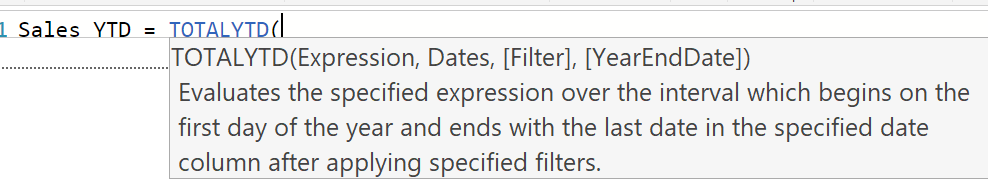The first two parameters are mandatory;

• Expression: The expression that applies aggregation of a value
• dates: The date field of the date dimension.

In our example, we are calculating the sum of the SalesAmount field in the FactInternetSales. So the expression would be: Sum(FactInternetSales[SalesAmount]).

For the date field, because we are using the default date dimension; when you type the date field name (in this case OrderDate from FactInternetSales), you will have the option to select a field from that table (remember that the date field is a date table behind the scene, Power BI creates the default date dimension for each date field) select the .[Date] field. This means the date field in the default date table.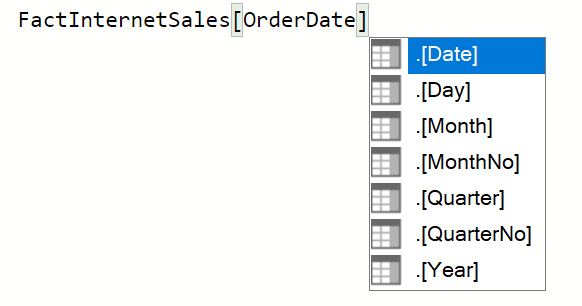So as a result, here is the expression to calculate year to date: (Note that this is a measure, not a column. To understand the difference between measure and calculated column read my https://radacad.com/measure-vs-calculated-column-the-mysterious-question-notarticle here)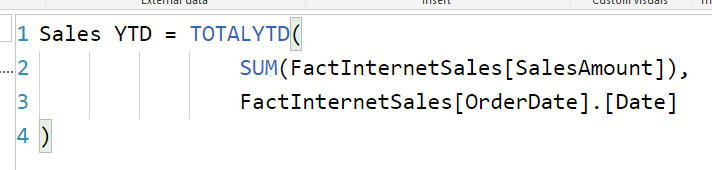and the sample output is: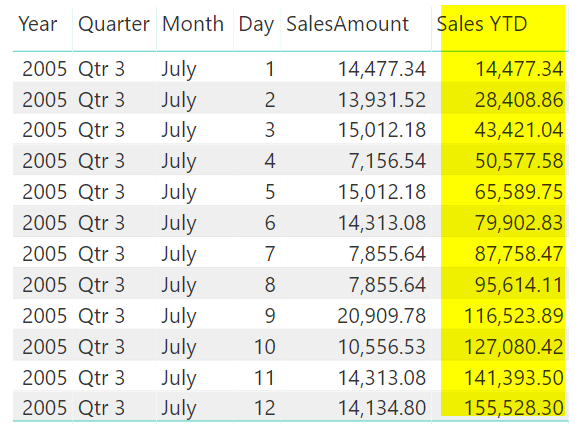As you can see, the Sales YTD measure is accumulated values of all dates before it from the start of the year (to get the right output, you have to have OrderDate in your visual, and it should be ordered by OrderDate ascending). The visual above shows calculation by every day, if we remove the day from the visual (you can remove it from the Fields section of the visual),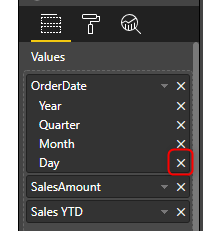then you can year to date calculation at the monthly level as below;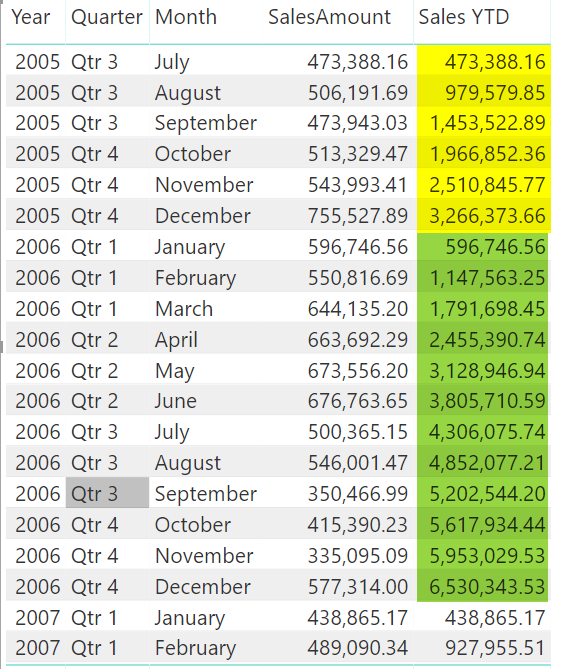As you can see in the screenshot above, the year to date calculation, for every month, is the accumulated sales of all months before that (from January of that year).

## Year to Date Another Approach: DatesYTD

TotalYTD is one of the methods of calculating the year to date value. There is another way, that can be helpful in more complex DAX expressions when you want to combine multiple filter criteria together. That way is using a function called DatesYTD combined with Calculate function. DatesYTD is a function that accepts only two parameters, which one of them is optional;

DatesYTD(<dates>, <year end date>)

DatesYTD returns a table as the output; a table with all dates in the period of a year to date. That is why for calculating the aggregation on that date range, it needs to be used with a function such as Calculate.

Here is how the calculation works with DatesYTD function;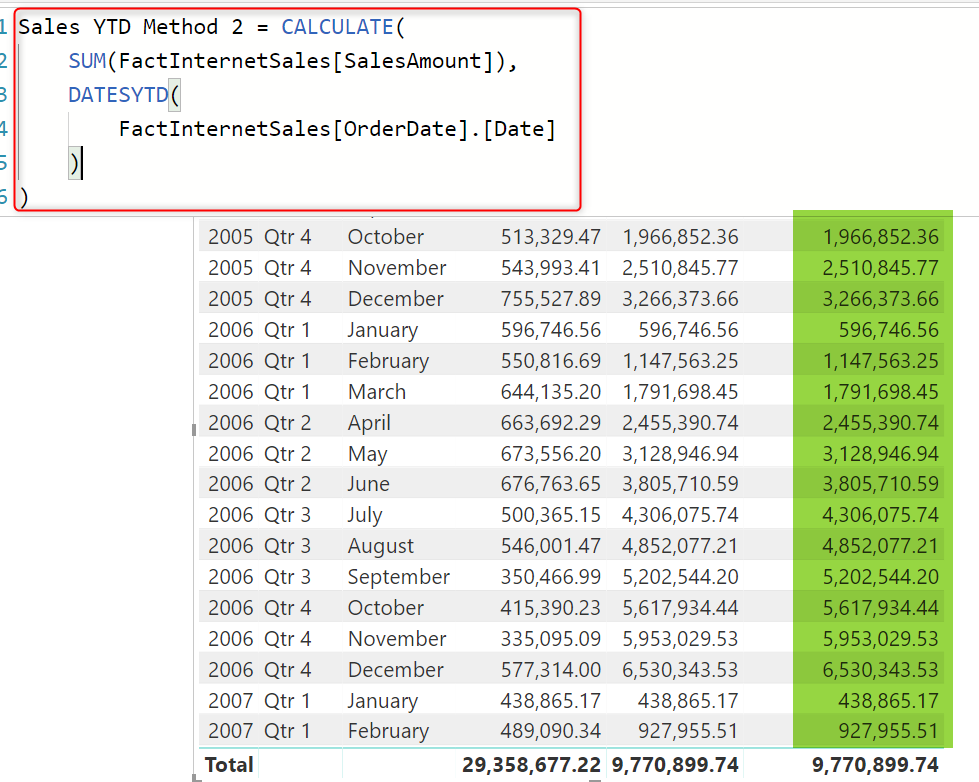You may ask which one is a preferred option? TotalYTD or DatesYTD. The answer depends on the type of filter you are using, If you are using multiple filter criteria, then I would suggest, DatesYTD, because when it is used inside a Calculate, then you can apply whatever filter you want. You may be able to do that still with TotalYTD, but you probably make the expression a bit complicated.

## Fiscal or Financial Year to Date

Calculating the calendar year to date was easy, how about the fiscal or financial calculation? do we have a function for those? No. However, there is a parameter that you can add in the expression and that makes the calculation fiscal. The <year end date> parameter is an optional parameter which we have not used in the previous example. When you do not assign a value for this parameter, the default value would be considered which is 31st of Dec of each year. If you want to specify a value for this parameter, this is an example of how you can do that:

As you can see the structure I provided the year-end date, was month/day. There are a few other options you can use as well, such as 06-30, 6/30, June 30, or 30 June. Anything that resolves a month/day scenario. It is, however, recommended to use month/day format. “06/30” value as the parameter here means that the end of the fiscal year is 30th of June of each year, and start as the result would be 1st of July of the year. Here is the output, as you can see the calculation restarts in July of each year instead of the calendar year to date which is starting from January.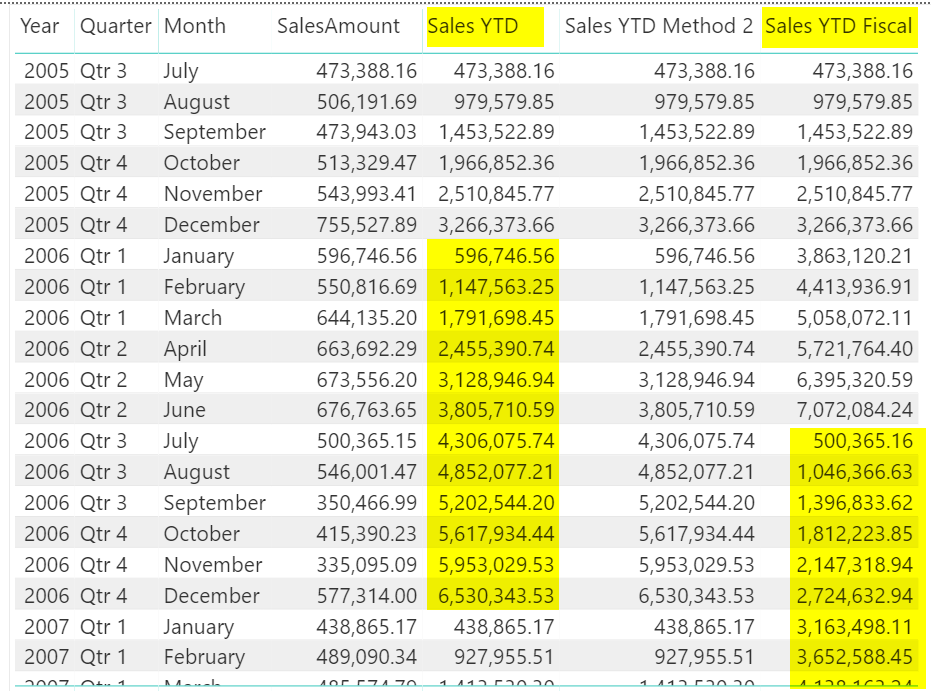The approach is very similar if you want to use DatesYTD approach, here is the code:

## Quarter to Date Calculation: TotalQTD

When you know how the calculation of the year to date works, you can guess how it would work for the quarter to date as well. The only difference is the function for the quarter to date, is called TotalQTD. It can be used exactly similar to the way that we used TotalYTD in the previous example;As you can see, this calculation, accumulate sales values up to the end of each quarter.

## Quarter to Date Calculation: DatesQTD

Similar to the DatesYTD, there is also a function for DatesQTD, which can be used exactly the same way. here is the code for it:

## Month to Date Calculation: TotalMTD

To calculate the month to date, you can use TotalMTD function very similar to the other functions you have seen in previous examples. here is the code:

And the output (note that you can test it better when you have DAY on your visual to see the accumulation happening);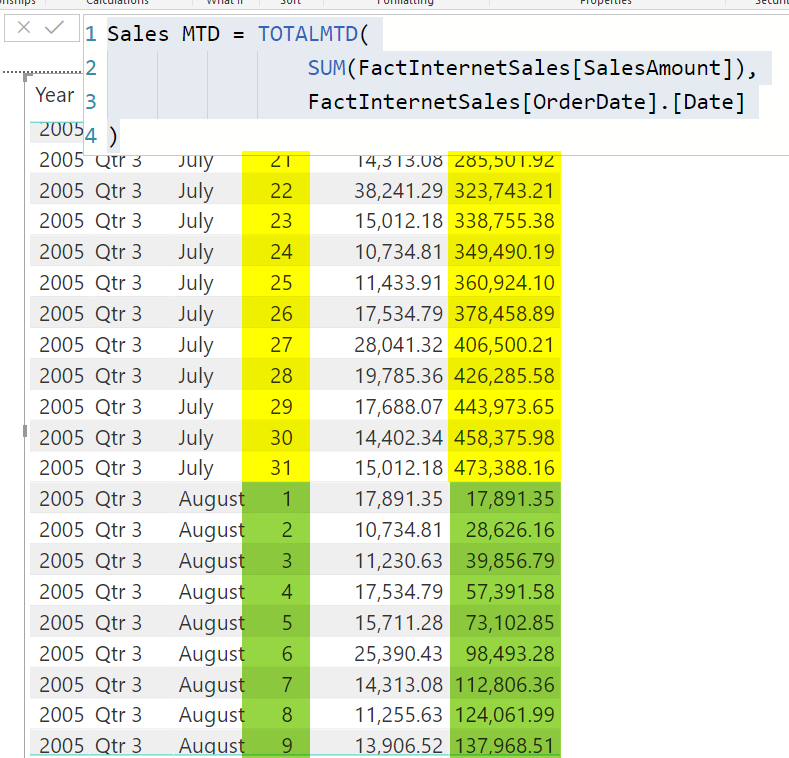## Month to Date Calculation: DatesMTD

The same approach can be applied for the DatesMTD as below;

## More Functions?

Well, as you have seen in this article, using Time intelligence functions is not hard to begin with. In the next article, I’ll explain a few other time intelligence functions. is there any particular function that you would like to learn about? let me know in the comments below and I’ll write about that too.

## Video:

Trainer, Consultant, Mentor
Reza Rad is a Microsoft Regional Director, an Author, Trainer, Speaker and Consultant. He has a BSc in Computer engineering; he has more than 20 years’ experience in data analysis, BI, databases, programming, and development mostly on Microsoft technologies. He is a Microsoft Data Platform MVP for nine continuous years (from 2011 till now) for his dedication in Microsoft BI. Reza is an active blogger and co-founder of RADACAD. Reza is also co-founder and co-organizer of Difinity conference in New Zealand.
His articles on different aspects of technologies, especially on MS BI, can be found on his blog: https://radacad.com/blog.
He wrote some books on MS SQL BI and also is writing some others, He was also an active member on online technical forums such as MSDN and Experts-Exchange, and was a moderator of MSDN SQL Server forums, and is an MCP, MCSE, and MCITP of BI. He is the leader of the New Zealand Business Intelligence users group. He is also the author of very popular book Power BI from Rookie to Rock Star, which is free with more than 1700 pages of content and the Power BI Pro Architecture published by Apress.
He is an International Speaker in Microsoft Ignite, Microsoft Business Applications Summit, Data Insight Summit, PASS Summit, SQL Saturday and SQL user groups. And He is a Microsoft Certified Trainer.
Reza’s passion is to help you find the best data solution, he is Data enthusiast.

## 2 thoughts on “Basics of Time Intelligence in DAX for Power BI; Year to Date, Quarter to Date, Month to Date”

•Mark says:

Really good article, especially the financial YTD when it is not based on a calendar year. How do you achieve the same for QTD based on a financial year. When I apply the filter for the year end it throws an error. The True/False expression does not specify a column,

•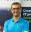Reza Rad says:

Hi Mark,
Correct, TotalQTD doesn’t have the year-end date parameter.
In most of the cases, because the fiscal quarter maps to normal quarters, this won’t create an issue anyways.
for example; a company that the fiscal year for them starts on the 1st of April. their first fiscal quarter would be from month 4 to 6, which is a calendar quarter itself.
you will have an issue in the calculation if the fiscal year starts on months which are not 1, 4, 7, 10. In these cases, you have two options. Write the logic of Fiscal YTD yourself. Or use a date dimension with a fiscal quarter column and do the calculation based on that.
Cheers
Reza